Related Articles

# Heavy Light Decomposition | Set 1 (Introduction)

• Difficulty Level : Hard
• Last Updated : 15 May, 2017

Heavy Light decomposition (HLD) is one of the most used techniques in competitive programming.

Example Problem: Let us understand Heavy-light decomposition (HLD) with the help of below example.

Suppose we have an unbalanced tree (not necessarily a Binary Tree) of n nodes, and we have to perform operations on the tree to answer a number of queries, each can be of one of the two types:

1. change(a, b): Update weight of the ath edge to b.
2. maxEdge(a, b): Print the maximum edge weight on the path from node a to node b. For example maxEdge(5, 10) should print 25.

Assume that nodes are numbered from 1 to n. There must be n-1 edges. Edge weights are natural numbers. Also assume that both type of queries are interspersed (approximately equal in number), and hence no type can be sidelined to compromise on complexity.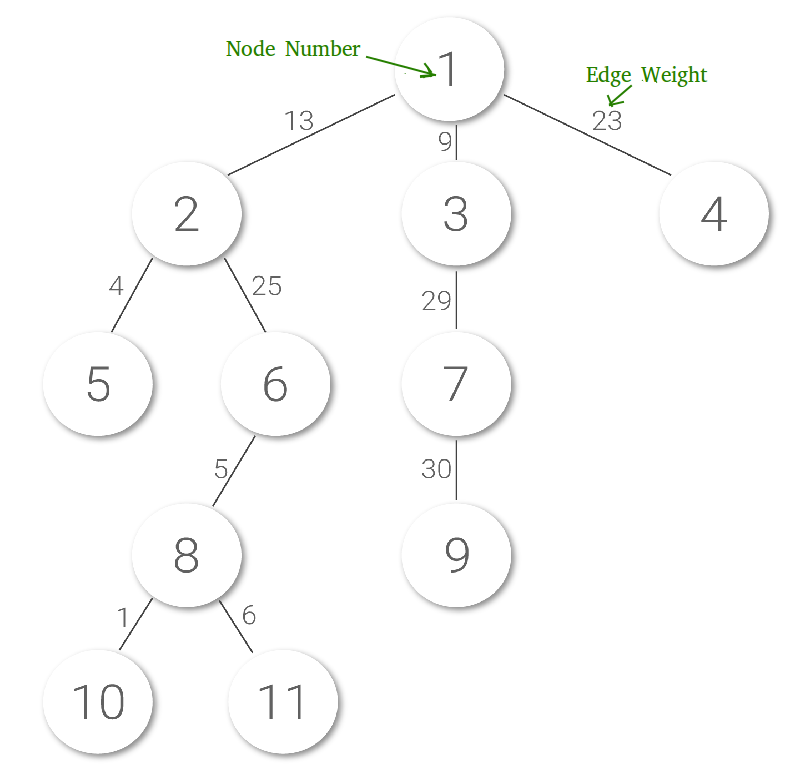Simple Solution: A Simple solution is to traverse the complete tree for any query. Time complexity of every query in this solution is O(n).

HLD Based Solution:

Upon careful observation of the two operations, we realize that we have seen them somewhere. Eureka! Segment Trees. A Segment Tree can be used to perform both types in O(log(n)). But wait! A Segment Tree can be built from a one-dimensional array / chain (set of nodes linked one after another), and what we have here is a tree. So, can we reduce the tree to chains?

The HLD based solution discussed in the post takes O(log2(n)) for maxEdge() and O(log n) for change().

Size of a node x is number of nodes in subtree rooted with the node x. Here is an image showing subtree sizes of each node written on top of them: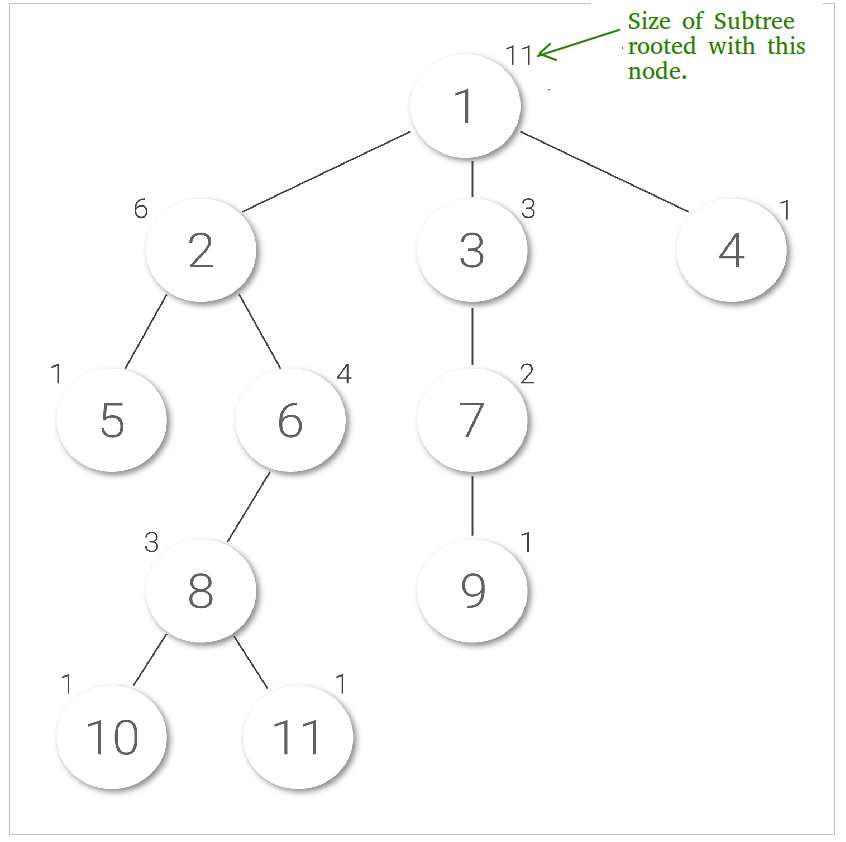HLD of a rooted tree is a method of decomposing the vertices of the tree into disjoint chains (no two chains share a node), to achieve important asymptotic time bounds for certain problems involving trees.

HLD can also be seen as ‘coloring’ of the tree’s edges. The ‘Heavy-Light’ comes from the way we segregate edges. We use size of the subtrees rooted at the nodes as our criteria.

An edge is heavy if size(v) > size(u) where u is any sibling of v. If they come out to be equal, we pick any one such v as special.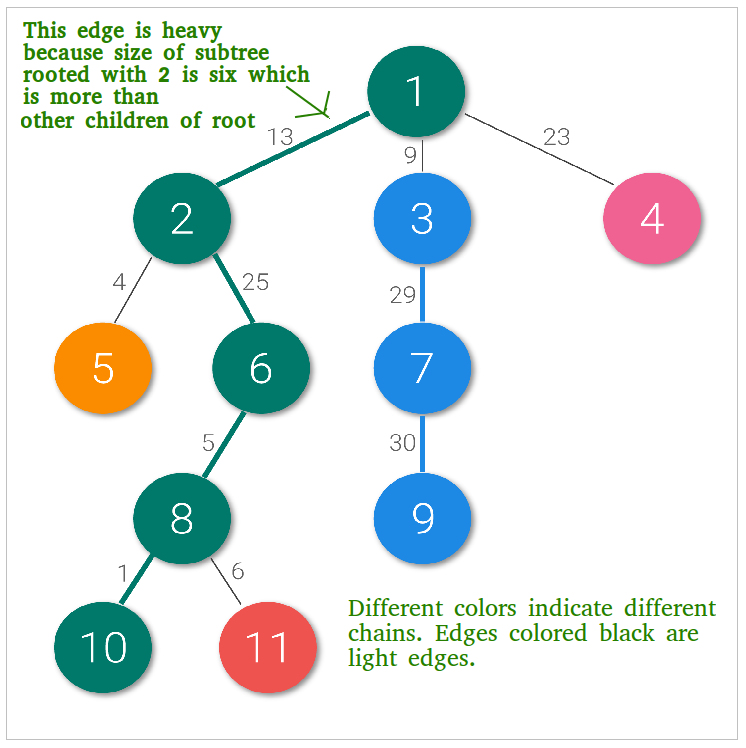change(u, v) operation:
Since Segment Tree is used as underlying data structure to represent individual chains, change is done using update of segment tree. So the time complexity of change operation is O(Log n).

maxEdge(u, v) operation:

1. We first find LCA of two nodes. Say node u is 11 and node v is 9. Their LCA is node 1.
2. Then we crawl up the tree from node u to the lca. If node u and lca belong to the same chain, we find the maximum from the range that represents the edges between them using segment tree. Else, we find the maximum from the chain to which u belongs, then change chains and repeat while we are not in the same chain.
3. We repeat the same step (as step 2) from v to lca and return maximum of two weights.

As per our example above, let’s take u as node 11 and v as node 9. LCA is node 1. We move from node 11 to node 1, and we change chains once. When we change chains, we shift from our queried node to the parent of head of the chain to which it belongs (11 changes to 8 here). Similarly node 9 to node 3 queried (including the light edge), and chain changed (node changed to 1).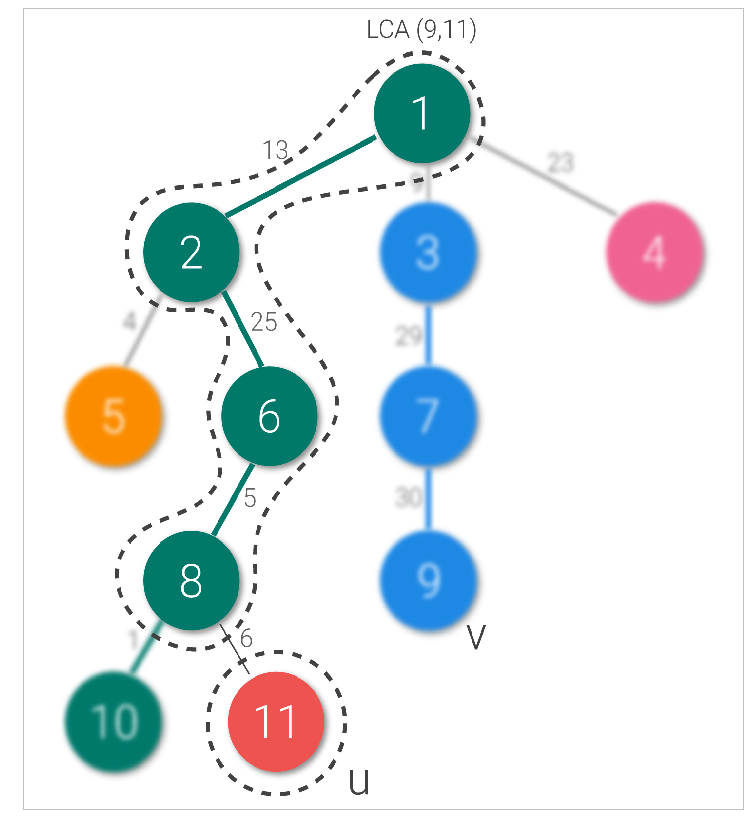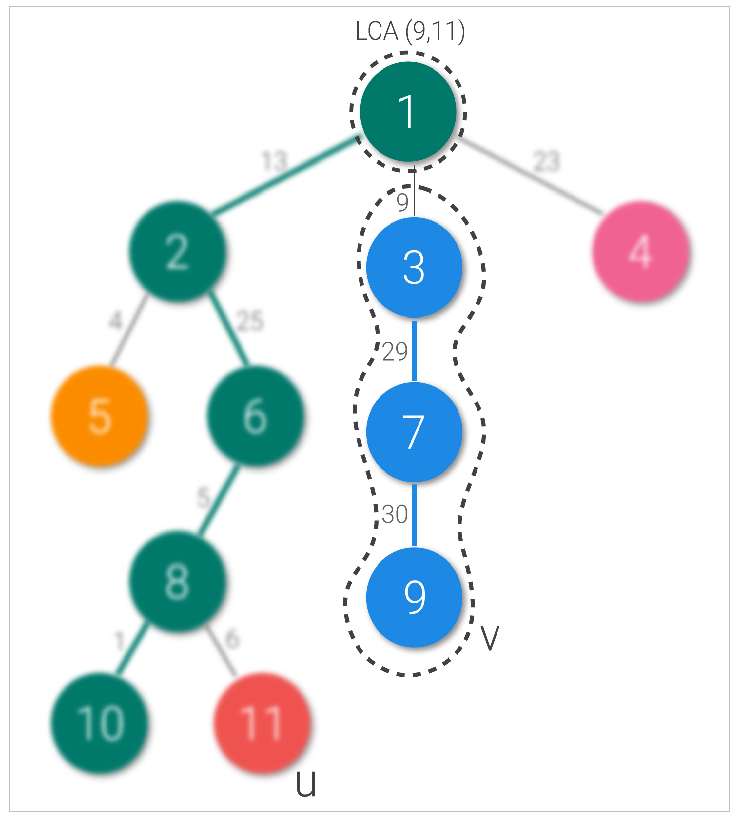Time Complexity of maxEdge is O(log2(n)). Querying maximum of a chain takes O(log(n)) time as chains are represented using Segment Tree and there are at-most O(log(n)) chains.

How is the number of chains O(log(n))?
All chains are connected by a light edge (see above examples). So the number of chains is bounded by number of light edges on any path. If we follow a light edge from the root, the subtree rooted at the resulting vertex has at most n/2 size. If we repeat, we land at a vertex with subtree size at most n/4, and so on. We conclude that number of light edges on any path from root to leaf is at most log(n) (Source: wcipeg)

The main idea of Heavy Light Decomposition of a unbalanced tree is to club vertices of long (or heavy) paths together in chains so that these linear chains can be queried in O(Log n) time using a data structure like Segment Tree.

In the next article, segment tree representation of chains in more detail and implementation of HLD solution for the problem is discussed as example.

Heavy Light Decomposition | Set 2 (Implementation)